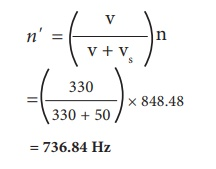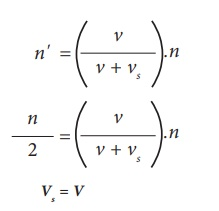Home | | Science 10th Std | Doppler Effect

# Doppler Effect

The whistle of a fast moving train appears to increase in pitch as it approaches a stationary listener and it appears to decrease as the train moves away from the listener.

DOPPLER EFFECT

The whistle of a fast moving train appears to increase in pitch as it approaches a stationary listener and it appears to decrease as the train moves away from the listener. This apparent change in frequency was first observed and explained by Christian Doppler (1803-1853), an Austrian Mathematician and Physicist. He observed that the frequency of the sound as received by a listener is different from the original frequency produced by the source whenever there is a relative motion between the source and the listener. This is known as Doppler effect This relative motion could be due to various possibilities as follows:

i.                     The listener moves towards or away from a stationary source

ii.                     The source moves towards or away from a stationary listener

iii.                     Both source and listener move towards or away from one other

iv.                     The medium moves when both source and listener are at rest

For simplicity of calculation, it is assumed that the medium is at rest. That is the velocity of the medium is zero.

Let S and L be the source and the listener moving with velocities vS and vL respectively. Consider the case of source and listener moving towards each other (Figure 5.7). As the distance between them decreases, the apparent frequency will be more than the actual source frequency.Let n and n' be the frequency of the sound produced by the source and the sound observed by the listener respectively. Then, the expression for the apparent frequency n' isHere, v is the velocity of sound waves in the given medium. Let us consider different possibilities of motions of the source and the listener. In all such cases, the expression for the apparent frequency is given in table 5.2.Suppose the medium (say wind) is moving with a velocity W in the direction of the propagation of sound. For this case, the velocity of sound, ‘v’ should be replaced with (v + W). If the medium moves in a direction opposite to the propagation of sound, then ‘v’ should be replaced with (v – W).

## Solved problems

1. A source producing a sound of frequency 90 Hz is approaching a stationary listener with a speed equal to (1/10) of the speed of sound. What will be the frequency heard by the listener?

Solution:

When the source is moving towards the stationary listener, the expression for apparent frequency is2. A source producing a sound of frequency 500 Hz is moving towards a listener with a velocity of 30 m s–1. The speed of the sound is 330 m s–1. What will be the frequency heard by listener?

Solution: When the source is moving towards the stationary listener, the expression for apparent frequency is3. A source of sound is moving with a velocity of 50 m s–1 towards a stationary listener. The listener measures the frequency of the source as 1000 Hz. what will be the apparent frequency of the source when it is moving away from the listener after crossing him? (velocity of sound in the medium is 330 m s–1)

Solution: When the source is moving towards the stationary listener, the expression for apparent frequency isn = 848.48 Hz.

The actual frequency of the sound is 848.48 Hz. When the source is moving away from the stationary listener, the expression for apparent frequency is= 736.84 Hz

4. A source and listener are both moving towards each other with a speed v/10 where v is the speed of sound. If the frequency of the note emitted by the source is f, what will be the frequency heard by the listener?

Solution: When source and listener are both moving towards each other, the apparent frequency is5. At what speed should a source of sound move away from a stationary observer so that observer finds the apparent frequency equal to half of the original frequency?

Solution: When the source is moving away from the stationary listener, the expression for the apparent frequency is## 1.Conditions for no Dopplereffect

Under the following circumstances, there will be no Doppler effect and the apparent frequency as heard by the listener will be the same as the source frequency.

·                 When source (S) and listener (L) both are at rest.

·                 When S and L move in such a way that distance between them remains constant.

·                 When source S and L are moving in mutually perpendicular directions.

·                 If the source is situated at the center of the circle along which the listener is moving.

## 2. Applications of Doppler effect

### (a) To measure the speed of an automobile

An electromagnetic wave is emitted by a source attached to a police car. The wave is reflected by a moving vehicle, which acts as a moving source. There is a shift in the frequency of the reflected wave. From the frequency shift, the speed of the car can be determined. This helps to track the over speeding vehicles.

### (b) Tracking a satellite

The frequency of radio waves emitted by a satellite decreases as the satellite passes away from the Earth. By measuring the change in the frequency of the radio waves, the location of the satellites is studied.

In RADAR, radio waves are sent, and the reflected waves are detected by the receiver of the RADAR station. From the frequency change, the speed and location of the aeroplanes and aircrafts are tracked.

###(d) SONAR

In SONAR, by measuring the change in the frequency between the sent signal and received signal, the speed of marine animals and submarines can be determined.

Tags : Conditions, Applications, Solved Example Problems , 10th Science : Chapter 5 : Acoustics
Study Material, Lecturing Notes, Assignment, Reference, Wiki description explanation, brief detail
10th Science : Chapter 5 : Acoustics : Doppler Effect | Conditions, Applications, Solved Example Problems